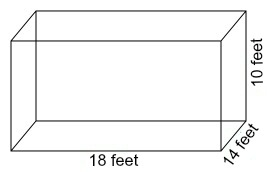# A room is 18 feet long and 14 feet wide with walls 10 feet high. If one can of paint covers 450...

## Question:

A room is 18 feet long and 14 feet wide with walls 10 feet high. If one can of paint covers 450 square feet, how many cans of paint would be needed in order to paint the four walls?

## Area of a Rectangle:

In mathematics, the area of a two-dimensional object is the amount of space inside the edges of that object. We have many formulas for the areas of different shapes in geometry. One of those formulas is for the rectangle, and it states that the area of a rectangle is equal to its length times its width.

To determine the number of cans of paint we would need to paint the four walls of this room, we need to determine the area of those four walls. Then, since one can of paint covers 450 square feet, we divide the area of the four walls by 450. The result will tell us how many cans of paint we will need to paint the four walls. First, let's find the area of the four walls. We will start by drawing a diagram of a room with a length of 18 feet, a width of 14 feet, and a height of 10 feet.We see that two of the walls of the room will be rectangles that are 18 feet by 10 feet, and the other two walls of the room will be rectangles that are 14 feet by 10 feet. The area formula for a rectangle gives that the area of one of the walls that is 18 feet by 10 feet is found by multiplying 18 by 10.

• 18 × 10 = 180

We get that two of the walls each have an area of 180 square feet. To find the area of one of the walls that is 14 feet by 10 feet, we multiply 14 by 10.

• 14 × 10 = 140

We get that the other two walls each have an area of 140 square feet. All together, we can determine the total area of all four walls by adding up each of their areas.

• 180 + 180 + 140 + 140 = 640

We get that the total area of the four walls of the room is 640 square feet. Now, we can determine the number of cans of paint needed by dividing 640 by 450.

• 640 ÷ 450 ≈ 1.4222

We get that we will need approximately 1.4222 cans of paint to paint the four walls of the room. Thus, we will need to buy 2 cans of paint to ensure that we will have enough.